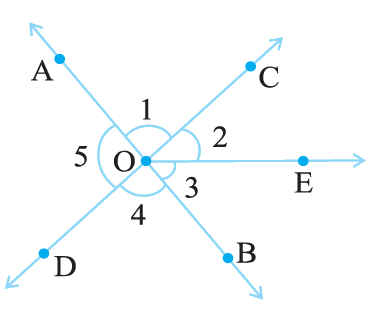# Ex.5.1 Q9 Lines and Angles - NCERT Maths Class 7

## Question

In the adjoining figure:

(i) Is $$\angle 1$$ adjacent to $$\angle 2$$ $$?$$

(ii) Is $$\angle AOC$$ adjacent to $$\angle AOE \,?$$

(iii) Do $$\angle COE$$ and $$\angle EOD$$ form a linear pair?

(iv) Are $$\angle BOD$$ and $$\angle DOA$$supplementary?

(v) Is $$\angle 1$$ vertically opposite to $$\angle 4\,?$$

(vi) What is the vertically opposite angle of $$\angle 5\,?$$Video Solution
Lines & Angles
Ex 5.1 | Question 9

## Text Solution

Steps:

(i) Yes, because they have common vertex $$O$$ and common arm $$OC.$$

(ii) No, they have non-common arms on either  side of common arms.

(iii) Yes, they form linear pair.

(iv) Yes, they are supplementary.

(v) Yes, they are vertical angles because they are formed due to intersection of straight lines.

(vi) Vertically opposite angle of $$\angle 5$$ is $$\angle 2 + \angle 3$$ i.e, $$\angle COB.$$

Learn from the best math teachers and top your exams

• Live one on one classroom and doubt clearing
• Practice worksheets in and after class for conceptual clarity
• Personalized curriculum to keep up with school